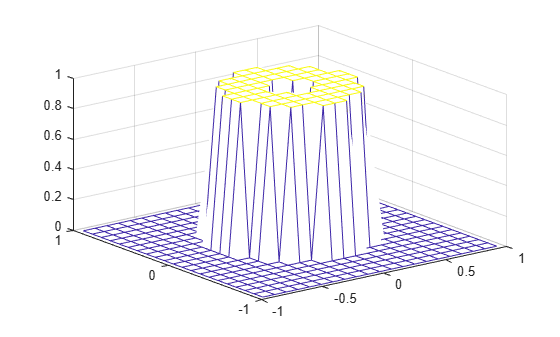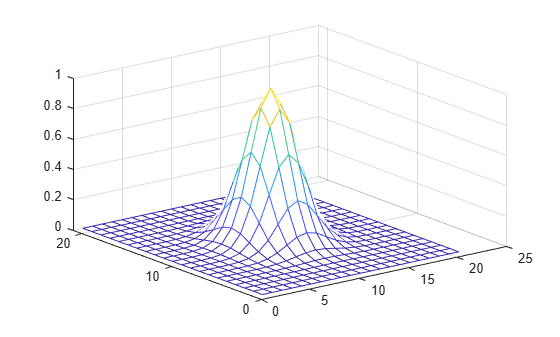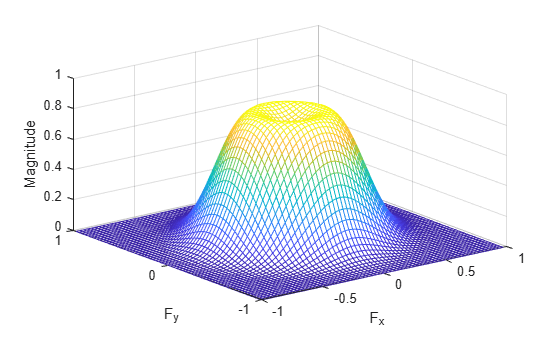# fwind2

2-D FIR filter using 2-D window method

## Syntax

``h = fwind2(Hd,win)``
``h = fwind2(f1,f2,Hd,win)``

## Description

The `fwind2` function designs 2-D FIR filters using the window method. `fwind2` uses a 2-D window specification to design a 2-D FIR filter based on the desired frequency response. `fwind2` works with 2-D windows only. Use `fwind1` to create a 2-D FIR filter from a 1-D window.

You can apply the 2-D FIR filter to images by using the `filter2` function.

example

````h = fwind2(Hd,win)` creates a 2-D FIR filter `h` by using an inverse Fourier transform of the desired frequency response `Hd` and multiplication by the window `win`.```
````h = fwind2(f1,f2,Hd,win)` lets you specify the desired frequency response `Hd` at arbitrary frequencies `f1` and `f2` along the x- and y-axes.```

## Examples

collapse all

This example shows how to design an approximately circularly symmetric two-dimensional bandpass filter using a 2-D window method.

Create the frequency range vectors `f1` and `f2` using `freqspace`. These vectors have length 21.

`[f1,f2] = freqspace(21,'meshgrid');`

Compute the distance of each position from the center frequency.

`r = sqrt(f1.^2 + f2.^2);`

Create a matrix `Hd` that contains the desired bandpass response. In this example, the desired passband is between 0.1 and 0.5 (normalized frequency, where 1.0 corresponds to half the sampling frequency, or $\pi$ radians).

```Hd = ones(21); Hd((r<0.1)|(r>0.5)) = 0;```

Display the ideal bandpass response.

```colormap(parula(64)) mesh(f1,f2,Hd)```Create a 2-D Gaussian window using `fspecial`. Normalize the window.

```win = fspecial('gaussian',21,2); win = win ./ max(win(:));```

Display the window.

`mesh(win)`Using the 2-D window, design the filter that best produces the desired frequency response

`h = fwind2(Hd,win);`

Display the actual frequency response of this filter.

`freqz2(h)`## Input Arguments

collapse all

Desired frequency response at equally spaced points in the Cartesian plane, specified as a numeric matrix. For accurate results, create `Hd` by using the `freqspace` function.

Data Types: `single` | `double` | `int8` | `int16` | `int32` | `int64` | `uint8` | `uint16` | `uint32` | `uint64`

2-D window, specified as a numeric matrix.

Data Types: `single` | `double`

Desired frequency along the x-axis. The frequency vector should be in the range [-1, 1], where 1.0 corresponds to half the sampling frequency, or π radians.

Data Types: `single` | `double`

Desired frequency along the y-axis. The frequency vector should be in the range [-1, 1], where 1.0 corresponds to half the sampling frequency, or π radians.

Data Types: `single` | `double`

## Output Arguments

collapse all

2-D FIR filter, returned as a numeric matrix of the same size as `win`.

Data Types: `double`

## Algorithms

`fwind2` computes `h` using an inverse Fourier transform and multiplication by the two-dimensional window `win`.

`${h}_{d}\left({n}_{1},{n}_{2}\right)=\frac{1}{{\left(2\pi \right)}^{2}}{\int }_{-\pi }^{\pi }{\int }_{-\pi }^{\pi }{H}_{d}\left({\omega }_{1},{\omega }_{2}\right){e}^{j{\omega }_{1}{n}_{1}}{e}^{j{\omega }_{2}{n}_{2}}d{\omega }_{1}d{\omega }_{2}$`
`$h\left({n}_{1},{n}_{2}\right)={h}_{d}\left({n}_{1},{n}_{2}\right)w\left({n}_{1},{n}_{2}\right)$`

 Lim, Jae S., Two-Dimensional Signal and Image Processing, Englewood Cliffs, NJ, Prentice Hall, 1990, pp. 202-213.